• #pragma GCC optimize("-Ofast","-funroll-all-loops") #include<bits/stdc++.h> //#define int long long using namespace std; const int N=4e5+10; int n,b[N],id[N],cnt,deg[N]; vector<...}c
• 数据结构与算法 ~ 查找 ~ 散列查找（哈希~线性探查法和二次探查法） /*search-hash*/ #include<math.h> #include<stdio.h> #include<stdlib.h> void Print(int *a){ int i; printf("\n"); ...
数据结构与算法 ~ 查找 ~ 散列查找（哈希~线性探查法和二次探查法）
/*search-hash*/
#include<math.h>
#include<stdio.h>
#include<stdlib.h>

void Print(int *a){
int i;
printf("\n");
for(i=1;i<=a;++i)
printf("%4d",a[i]);
}/*Print*/

int formatlist(){
int e;
printf("\n====创建哈希表========");
printf("\n1-线性探查法 ");
printf("\n2-二次探查法");
printf("\n0-退出");
printf("\n请你选择:");
scanf("%d",&e);
return e;
}/*formatlist*/

void hashprint(int *hashtable){
int i;
/*打印哈希表*/
for(i=0;i<19;++i){
if(hashtable[i]!=-1)
printf("\n[%d]=%d",i,hashtable[i]);
}
}/*hashprint*/

/*构造哈希表*/
void createhash(int *hashtable,int *collision){
int i ,e,hkey,d,k=1;
/*哈希表初始化*/
for (i=0;i<19;++i)
hashtable[i]=-1;
printf("\n请输入数值(exit for 0):");
scanf("%d",&e);
while(e!=0&&k<=19){
hkey=e%13;
d=hkey;i=1;
while(hashtable[d]!=-1){
if (hashtable[d]!=e && i<=collision)
d=(hkey+collision[i++])%19;
else if (hashtable[d]==e){
printf("数据重复!");
k--;
break;
}else if(i>collision){
printf("冲突次数太多!");
exit(0);
}
}/*while*/
if (hashtable[d]==-1)
hashtable[d]=e;
printf("\n请输入数值(exit for 0):");
scanf("%d",&e);
k++;
}/*while*/
if (k>19)
printf("空间满了!");  /*输入的数据超过所分配的空间*/
}/*createhash*/

/*哈希表中进行查找*/
int  searchhash(int *hashtable,int *collision,int e){
int hkey,d,i;
hkey=e%13;
d=hkey;i=1;
while(hashtable[d]!=e && hashtable[d]!=-1 && i<=collision)
d=(hkey+collision[i++])%19;
if (hashtable[d]==e ){
printf("\n数据：%d=被找到 , 地址： [%d],t探测次数：%d. ",e,d,i);
return 1;
}else {
printf("\n数据没有找到.\n");
return 0;
}
}/*search*/

/*插入哈希表中*/
void hashinsert(int *hashtable,int *collision,int e){
int i,hkey,d;
hkey=e%13;
d=hkey;i=1;
while(hashtable[d]!=-1){
if (hashtable[d]!=e && i<=collision)
d=(hkey+collision[i++])%19;
else if(i>collision){
printf("冲突次数太多!");
exit(0);
}
}/*while*/
if (hashtable[d]==-1){
hashtable[d]=e;
printf("\n元素被插入!");
hashprint(hashtable);
}
}/*createhash*/

int main(){
int hashtable, prode,secondprode;
int i,j=1;
int k=0,e,values;
/*线形探测再散列增量表初始化*/
for (i=1;i<=18;++i)
prode[i]=i;
prode=i-1;
/*二次线形探测再散列增量表初始化*/
for(i=1;i<=18&&j<=9;++i){
secondprode[i]=pow((double)(-1),(i+1)) * pow((double)(j),2);
k++;
if (k==2) {
j++;k=0;
}
}
secondprode=i-1;
Print(prode);
Print(secondprode);
while(1){
e=formatlist();
if (e==0)
break;
switch(e){
case 1:{/*用线形探测再散列构造哈希表*/
createhash(hashtable,prode);
break;
}
case 2:{/*用二次线形探测再散列构造哈希表*/
createhash(hashtable,secondprode);
break;
}
}/*switch*/
hashprint(hashtable);
while(1){
printf("\n请输入你想查找的数据(exit for -1):");
scanf("%d",&values);
if (values==-1) break;
switch(e){
case 1:
if (!searchhash(hashtable,prode,values))   /*哈希表查找*/
hashinsert(hashtable,prode,values);      /*查找不成功则插入该元素*/
break;
case 2:
if (!searchhash(hashtable,secondprode,values))
hashinsert(hashtable,secondprode,values);  /*用二次线形探测再散列构造哈希表*/
break;
default:
printf("你的输入错误!");
}/*switch*/
}/*while*/
}/*while*/
system("pause");
exit(0);
}/*main*/
运行结果：

1   2   3   4   5   6   7   8   9  10  11  12  13  14  15  16  17  18
1  -1   4  -4   9  -9  16 -16  25 -25  36 -36  49 -49  64 -64  81 -81
====创建哈希表========
1-线性探查法
2-二次探查法
0-退出
请你选择:1

请输入数值(exit for 0):26

请输入数值(exit for 0):36

请输入数值(exit for 0):41

请输入数值(exit for 0):38

请输入数值(exit for 0):44

请输入数值(exit for 0):15

请输入数值(exit for 0):68

请输入数值(exit for 0):12

请输入数值(exit for 0):6

请输入数值(exit for 0):51

请输入数值(exit for 0):25

请输入数值(exit for 0):0

=26
=41
=15
=68
=44
=6
=36
=38
=12
=51
=25
请输入你想查找的数据(exit for -1):25

数据：25=被找到 , 地址： ,t探测次数：4.
请输入你想查找的数据(exit for -1):26

数据：26=被找到 , 地址： ,t探测次数：1.
请输入你想查找的数据(exit for -1):41

数据：41=被找到 , 地址： ,t探测次数：1.
请输入你想查找的数据(exit for -1):68

数据：68=被找到 , 地址： ,t探测次数：2.
请输入你想查找的数据(exit for -1):44

数据：44=被找到 , 地址： ,t探测次数：1.
请输入你想查找的数据(exit for -1):6

数据：6=被找到 , 地址： ,t探测次数：1.
请输入你想查找的数据(exit for -1):36

数据：36=被找到 , 地址： ,t探测次数：1.
请输入你想查找的数据(exit for -1):38

数据：38=被找到 , 地址： ,t探测次数：1.
请输入你想查找的数据(exit for -1):12

数据：12=被找到 , 地址： ,t探测次数：2.
请输入你想查找的数据(exit for -1):51

数据：51=被找到 , 地址： ,t探测次数：3.
请输入你想查找的数据(exit for -1):

展开全文数据结构与算法 查找 散列查找 哈希
• 已知一组关键字为(39，49，54，38，44，28，68，12，06，77)，用除余法构造散列函数，用线性探查法解决冲突构造这组关键字的散列表。 　解答:为了减少冲突，通常令装填因子α<l。这里关键字个数n=10，不妨取m=13...
以下是用线性探测法构造哈希表的一个具体例子： 已知一组关键字为(39，49，54，38，44，28，68，12，06，77)，用除余法构造散列函数，用线性探查法解决冲突构造这组关键字的散列表。 　　解答:为了减少冲突，通常令装填因子α<l。这里关键字个数n=10，不妨取m=13，此时α≈0.77，散列表为T[0..12]，散列函数为：h(key)=key％13。     　由除余法的散列函数计算出的上述关键字序列的散列地址为(0，10，2，12，5，2，3，12，6，12)。     　前5个关键字插入时，其相应的地址均为开放地址，故将它们直接插入T，T[10)，T，T和T中。     　当插入第6个关键字28时，其散列地址2(即h(28)=28％13=2)已被关键字54(28和54互为同义词)占用。故探查h1=(2+1)％13=3，此地址开放，所以将15放入T中。     　当插入第7个关键字68时，其散列地址3已被非同义词28先占用，故将其插入到T中。     　当插入第8个关键字12时，散列地址12已被同义词38占用，故探查hl=(12+1)％13=0，而T亦被26占用，再探查h2=(12+2)％13=1，此地址开放，可将12插入其中。
类似地，第9个关键字06直接插入T中；而最后一个关键字77插人时，因探查的地址12，0，1，…，6均非空，故51插入T中。
展开全文• 【项目一 - 验证算法之线性探查法】 /* *烟台大学计算机与控制工程学院 *作 者：张雨萌 *完成日期：2017年11月30日 */  问题描述：认真阅读并验证哈希表实施查找的相关算法，写程序建立序列{16, 74, 60,...
【项目一 - 验证算法之线性探查法】

/*
*烟台大学计算机与控制工程学院
*作    者：张雨萌
*完成日期：2017年11月30日

*/     

问题描述：认真阅读并验证哈希表实施查找的相关算法，写程序建立序列{16, 74, 60, 43, 54, 90, 46, 31, 29, 88, 77}的哈希                                       表，装填因子定为0.8，哈希函数为h(k)=key%p，p=11，采用线性探查法解决冲突。
程序功能：（1）输出建立的哈希表；
（2）完成关键字为29的元素的查找；
（3）在上述哈希表中删除关键字为77的元素，再显示哈希表。
程序及代码：

#include <stdio.h>

#define MaxSize 100         //定义最大哈希表长度
#define NULLKEY -1          //定义空关键字值
#define DELKEY  -2          //定义被删关键字值

typedef int KeyType;        //关键字类型
typedef char * InfoType;    //其他数据类型
typedef struct
{
KeyType key;            //关键字域
InfoType data;          //其他数据域
int count;          //探查次数域
} HashData;

typedef HashData HashTable[MaxSize];        //哈希表类型

void InsertHT(HashTable ha,int &n,KeyType k,int p)  //将关键字k插入到哈希表中
{
{
}
else                    //发生冲突时采用线性探查法解决冲突
{
i=1;                //i记录x[j]发生冲突的次数
do
{
i++;
}
}
n++;
}
void CreateHT(HashTable ha,KeyType x[],int n,int m,int p)  //创建哈希表
{
int i,n1=0;
for (i=0; i<m; i++)         //哈希表置初值
{
ha[i].key=NULLKEY;
ha[i].count=0;
}
for (i=0; i<n; i++)
InsertHT(ha,n1,x[i],p);
}
int SearchHT(HashTable ha,int p,KeyType k)      //在哈希表中查找关键字k
{
{
i++;                //采用线性探查法找下一个地址
}
else                    //查找失败
return -1;
}
int DeleteHT(HashTable ha,int p,int k,int &n)   //删除哈希表中关键字k
{
{
n--;            //哈希表长度减1
return 1;
}
else                //在哈希表中未找到该关键字
return 0;
}
void DispHT(HashTable ha,int n,int m)    //输出哈希表
{
float avg=0;
int i;
printf(" 哈希表地址:\t");
for (i=0; i<m; i++)
printf(" %3d",i);
printf(" \n");
printf(" 哈希表关键字:\t");
for (i=0; i<m; i++)
if (ha[i].key==NULLKEY || ha[i].key==DELKEY)
printf("    ");         //输出3个空格
else
printf(" %3d",ha[i].key);
printf(" \n");
printf(" 搜索次数:\t");
for (i=0; i<m; i++)
if (ha[i].key==NULLKEY || ha[i].key==DELKEY)
printf("    ");         //输出3个空格
else
printf(" %3d",ha[i].count);
printf(" \n");
for (i=0; i<m; i++)
if (ha[i].key!=NULLKEY && ha[i].key!=DELKEY)
avg=avg+ha[i].count;
avg=avg/n;
printf(" 平均搜索长度ASL(%d)=%g\n",n,avg);
}
int main()
{
int x[]= {16,74,60,43,54,90,46,31,29,88,77};
int n=11,m=13,p=11,i,k=29;
HashTable ha;
CreateHT(ha,x,n,m,p);
printf("\n");
DispHT(ha,n,m);
i=SearchHT(ha,p,k);
if (i!=-1)
printf(" ha[%d].key=%d\n",i,k);
else
printf(" 未找到%d\n",k);
k=77;
printf(" 删除关键字%d\n",k);
DeleteHT(ha,p,k,n);
DispHT(ha,n,m);
i=SearchHT(ha,p,k);
if (i!=-1)
printf(" ha[%d].key=%d\n",i,k);
else
printf(" 未找到%d\n",k);
printf(" 插入关键字%d\n",k);
InsertHT(ha,n,k,p);
DispHT(ha,n,m);
printf("\n");
return 0;
}
            运行结果：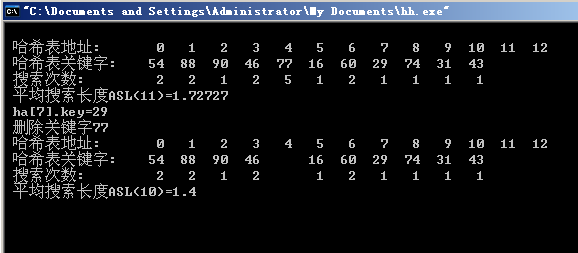知识点总结：学习了哈希表的建立算法，学习了线性探查法的思路，及在哈希表中删除元素的算法
心得体会：了解了线性探查法的过程，可以自主画出流程图，算法的实现加深了对该法的理解程度。
展开全文• 一、线性探查法 散列表基础介绍与散列冲突见文章：https://blog.csdn.net/qq_41453285/article/details/103517420 线性探查法的基本思想：在产生散列冲突时，将产生散列冲突的关键字向后存储 当使用了线性探查法...
本文代码下载：
一、线性探查法
散列表基础介绍与散列冲突见文章：https://blog.csdn.net/qq_41453285/article/details/103517420线性探查法的基本思想：在产生散列冲突时，将产生散列冲突的关键字向后存储当使用了线性探查法之后，整个散列表就被视为一个环形表了
二、图示说明
散列函数为f(k)=k%11。且散列表的当前状态如下图所示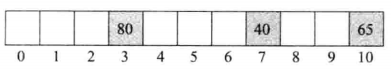当要插入58关键字时，求得其起始桶为3=58%11，但是3号桶已经存放了80，那么就将其插入到4的索引处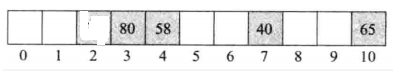此时再插入一个关键字24，其起始桶为2=24%11，那么直接插入桶2处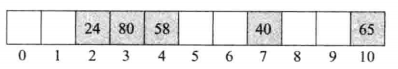当再要插入35时，求得其起始桶为2=35%11，但是桶2已经有元素了，那就向后移动，一直移动到桶5处才有地方存储，那么就存储在桶5处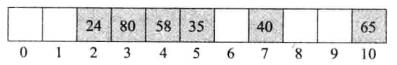这就是线性探查法的基本思想
三、线性探查法下关键字的增加、查询、删除

增加：
就是上面图示说明所介绍的思想

查询：
1.首先根据要查找的关键字k，首先搜索起始桶f(k)，如果起始桶就是要查找的关键字就退出(查找的关键字不存在)；如果起始桶为空就退出；如果起始桶不为空，说明产生了散列冲突就进行下一步2.接着向后面遍历，如果查找到了就返回；如果再向后遍历的时候遇到了空桶就退出3.如果向后遍历的时候循环遍历又再次回到起始桶f(k)还是没有找到就退出(查找的关键字不存在)

删除：
删除一个关键字需要删除之后保证查询操作可以正常进行，例如下面如果删除了关键字58，那么35这个关键字就永远也找不到了，因为删除58之后，桶4被置位空。当查找35的时候首先查找桶2、再对比桶3、再对比桶4，发现桶4为空，那么就退出查找（此时35显示未查找到，实际上存在于散列表中）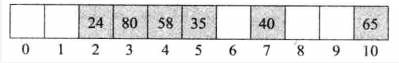删除的方法①：删除之后，从删除的下一个桶开始，逐个检查每个桶，以确定要移动的元素，直到达到一个空桶或者回到删除位置为止就退出移动操作（但是需要注意，不要把一个数对移到它的起始桶之前，否则对这个数的查找就可能失败）。例如删除下面的58之后，就要把35移到桶4处，其余元素不动删除的方法②：为每个桶增加一个域neverUsed，思想如下：
在散列表初始化时，每个域被置位true。当一个关键字被插入桶之后，域neverUsed被置为false。当关键字被删除之后，域neverUsed也不会被重新置为true在增加了域neverUsed的散列表中，查找元素在遇到空桶时不会退出查找，而是遇到一个桶为true时才退出。因此删除一个散列表的元素之后，不需要考虑移动元素了，因为查找操作遇到空桶但是桶的域neverUsed为false还继续会向后查找为了提高性能，如果桶中的所有或大多数桶的域neverUsed变为false之后，搜索操作可能会失败。因此当很多桶的域neverUsed变为false之后，就必须重新组织这个散列表

四、随机探查分析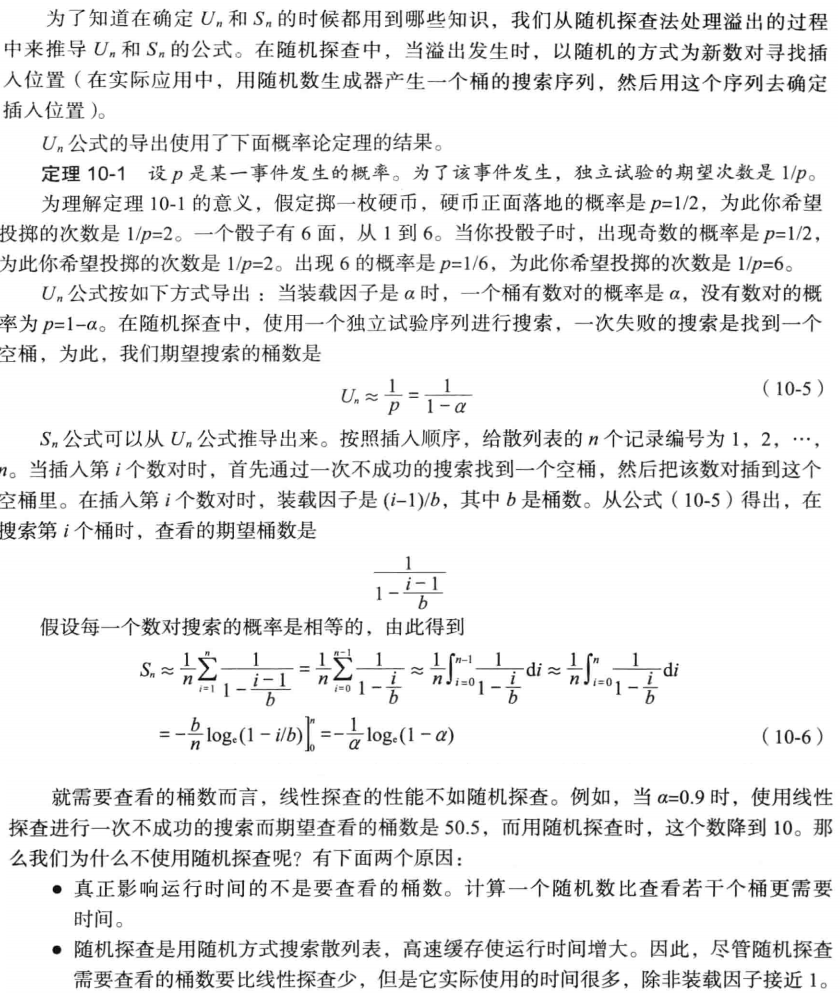五、选择一个除数D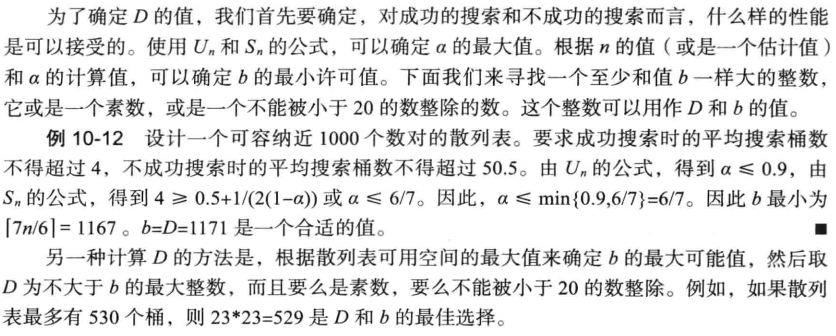六、编码实现

头文件定义
#include <iostream>
#include <string>

using std::cout;
using std::endl;
using std::cin;
using std::string;
using std::pair;

异常类的处理
哈希表满时抛出
class hashTableFull
{
public:
hashTableFull(std::string theMessage ="The hash table is full")
{
message = theMessage;
}
const char* what() {
return message.c_str();
}
private:
std::string message;
};

关键字映射整数模板类
如果插入的关键字为字符串，那么就需要把关键字映射为一个整数，然后运用散列函数求得起始桶，然后进行插入下面建立了一系列的模板类，用来针对传入的关键字数据类型，将其转换为一个非负的整数，然后运用于散列函数详细介绍也可以参见文章中的七：https://blog.csdn.net/qq_41453285/article/details/103517420
template<class K> class hash;

template<>
class hash<std::string>
{
public:
std::size_t operator()(const std::string theKey)const
{
unsigned long hashValue = 0;
int length = (int)theKey.length();
for (int i = 0; i < length; i++) {
hashValue = hashValue * 5 + theKey.at(i);
}

return std::size_t(hashValue);
}
};

template<>
class hash<int>
{
public:
std::size_t operator()(const int theKey) const
{
return std::size_t(theKey);
}
};

template<>
class hash<long>
{
public:
std::size_t operator()(const long theKey) const
{
return std::size_t(theKey);
}
};

类定义
template<class K,class E>
class hashTable
{
public:
hashTable(int theDivisor = 11);
~hashTable();

bool empty()const;
int size()const;
std::pair<const K, E>* find(const K& theKey)const;
void insert(const std::pair<const K,E>& thePair);
void output(std::ostream& out)const; //打印散列表
private:
int search(const K& theKey)const;
private:
std::pair<const K, E>** table; //散列表
hash<K> hash; //把类型K映射为一个整数
int dSize;    //字典中的数对个数
int divisor;  //散列函数除数
};

构造函数
template<class K, class E>
hashTable<K, E>::hashTable(int theDivisor = 11)
{
this->divisor = theDivisor;
this->dSize = 0;

//将整个散列表置空
this->table = new std::pair<const K, E>*[theDivisor];
for (int i = 0; i < theDivisor; ++i)
this->table[i] = nullptr;
}
析构函数
template<class K, class E>
hashTable<K, E>::~hashTable()
{
delete[] this->table;//释放数组
this->table = nullptr;
}
empty()、size()函数
template<class K, class E>
bool hashTable<K, E>::empty()const
{
return (this->dSize == 0);
}

template<class K, class E>
int hashTable<K, E>::size()const
{
return this->dSize;
}

search函数
这个函数返回一个桶序号b，有3中用途
①table[b]是一个指针，指向关键字为theKey的数对②散列表没有关键字为theKey的数对，且table[b]=nullptr，则可以把关键字theKey插入散列表③散列表没有关键字为theKey的数对，但table[b]!=nullptr，table[b]!=theKey，表示表已满
template<class K, class E>
int hashTable<K, E>::search(const K& theKey)const
{
int i = ((int)this->hash(theKey) / this->divisor);
int j = i;

//循环遍历
do {
//如果桶的关键字为要查找的关键字或者桶为空退出，返回桶索引
if ((this->table[j]->first == theKey) || (this->table[j] == nullptr))
return j;
j = ((j + 1) % this->divisor);
} while (j != i);

return j;
}

find函数
template<class K, class E>
std::pair<const K, E>* hashTable<K, E>::find(const K& theKey)const
{
int b = this->search(theKey);

//没有查找到
if ((this->table[b] == nullptr) || this->table[b]->first != theKey)
return nullptr;

//查找到，返回
return this->table[b];
}

insert函数
template<class K, class E>
void hashTable<K, E>::insert(const std::pair<const K, E>& thePair)
{
int b = this->search(thePair.first);

//如果为空就插入
if (this->table[b] == nullptr) {
this->table[b] = new std::pair<const K, E>(thePair);
this->dSize++;
}
else{
//如果关键字与插入的关键字相同，更新至
if (this->table[b]->first == thePair.first)
this->table[b]->second = thePair.second;
else//满了，抛出异常
throw hashTableFull();
}
}

主函数
int main()
{
hashTable<int,int>* myHashTable = new hashTable<int, int>(11);

myHashTable->insert(std::pair<int,int>(80,1));
myHashTable->insert(std::pair<int, int>(40, 2));
myHashTable->insert(std::pair<int, int>(65, 3));

myHashTable->output(std::cout);
std::cout << std::endl;

myHashTable->insert(std::pair<int, int>(58, 4));
myHashTable->insert(std::pair<int, int>(24, 5));

myHashTable->output(std::cout);
std::cout << std::endl;

myHashTable->insert(std::pair<int, int>(35, 5));

myHashTable->output(std::cout);
std::cout << std::endl;

return 0;
}
:前为索引号，:后为关键字值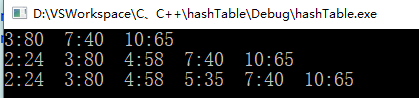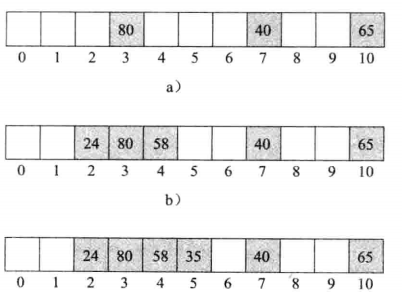性能分析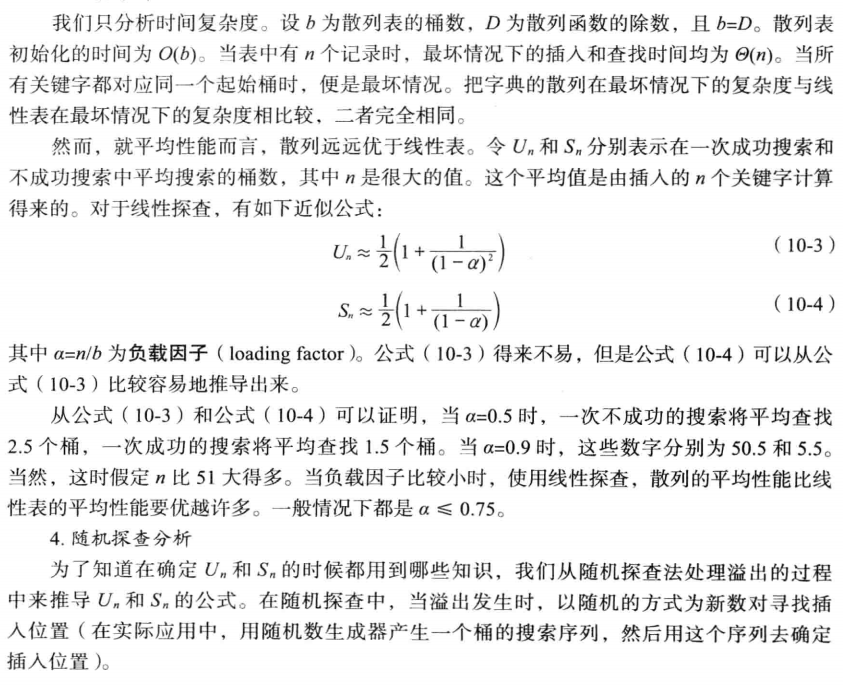展开全文•  * 用开放地址法中的线性探查法解决冲突实现哈希表的运算。  */ public class MyHashSearch {  public static final int SIZE = 10;  public static final int NULLKEY = -1;  public sta算法 数据
• 6-3 哈希表的创建及查找(线性探查法) (10分) 实现哈希表创建及查找算法，哈希函数使用除余法，用线性探测法处理冲突。 函数接口定义： void CreateHash(HashTable HT[],int n); //输入不大于m的n个不为0（0表示空值...数据结构 哈希表
• E - 线性探查法 按照哈希的操作进行逆操作，求出每一位最小数值，利用set维护压入的最小值。 #include&lt;stdio.h&gt; #include&lt;bits/stdc++.h&gt; using namespace std; typedef long long ll; ...
• 散列表中条目数的比例较小时，使用线性探查法的效率较高. [code="java"]package Hash; import java.nio.BufferOverflowException; /** * 散列表的设计 * 线性探查法(linear probing) * @...java
• 在大学里选修过数据结构的同学大部分都知道 hashhashhash 算法的线性探查法： 假设有一个元素互不相同的正整数数组 a[1…n]a[1\ldots n]a[1…n]，我们用以下方法得到数组 b[1…n]b[1\ldots n]b[1…n]： 初始时 b[i]b...拓扑排序
• 有两种常见的碰撞处理的方法，分别是链地址线性探测。 1 链地址 链地址：将大小为M的数组中的每个元素指向一条结点类型的链表，链表中保存散列值为该元素的索引的键值对。 在一张含...
• HashTable.js function getPrime(n) { if (n ) { return n; } var isPrime = false; while (!isPrime) { isPrime = true; var sqrt = Math.ceil(Math.sqrt(n));...再散列法和平方探查法略散列表
• 数据结构基本的操作，作用不大，但是思路清晰，值得一看！散列表
• 【题目】已知散列表的地址空间为A[0..10]，散列函数H(k)=k mod 11，采用线性探查法处理冲突。将下列数据{24, 15, 38, 46, 79, 82, 52, 39, 85, 143, 231}依次插入到散列表中，请写出散列表的结果，并计算出在等概率...经验分享
• 先将一组数据以除留余存储，就是说 假定一个线性表为a[]={18, 75, 60, 43, 54, 90, 46}，选取散列函数为：h(K)=K%m 取m=13 得到的 h(K) 就是数据在 storeArray[ ] 中存储的下标 例： a[]={18, 75, 60, 43, 54, ...数据结构 算法
• #include #include using namespace std; const int P = 7; const int DefaultSize = 7; enum KindOfStatus{Active,Empty,Deleted}; class HashTable ... HashTable(const int d,int sz = DefaultSize) {iostream namespace enum
• const int DefaultSize = 100;enum KindofStatus {Active, Empty, Deleted};template<class E, class K>class HashTable { public: HashTable(int d, int sz = DefaultSize); ~HashTabl...
• 【哈希表 | 散列表】根据关键字的值来计算出关键字在表中的地址 ...开放地址 链地址 查找失败的次数 装填因子 举例 构造 【问题举例】 1. 关键字序列为{7, 4, 1, 14, 10...哈希表 散列表
• #include "iostream.h"const int m=13;const int n=10;int r[n+1];class lnode{public:int j; int h(int k) //构造散列函数 { return k%13; } void creat(int ht[m],int n)c++ null include class
• （1）哈希函数采用除留余数法，解决哈希冲突方法采用开放定址法的线性探查法。 （2）设计函数表构造头文件。头文件包括节点结构体定义，以及哈希表初始化、哈希表元素插入、哈希表元素删除、哈希表查找和哈希表撤销...
•数据结构
• 题解 b数组一定有数值%n==位置的元素 这个是无碰撞直接插入的元素 使用set维护所有当前插入的元素 所有无碰撞的元素直接加入set 每次取出数值最小的元素插入 插入后会影响到后面第一个空闲位置 检测他出现的位置和......